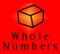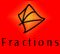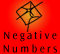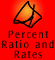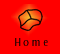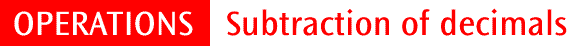| Quick Quiz |

Subtraction of decimals is very similar to subtraction of whole numbers. It is important to understand the place value of each digit, both in performing the operation and in determining which zeros are important.

Example 1: Using LAB to demonstrate 1.65 - 0.78 = 0.87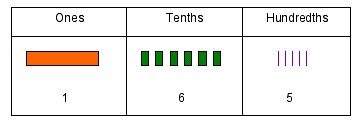I have 1.65 and I am subtracting 0.78 from this. I need to start with the hundredths. 5 minus 8 I cannot do, so I need to rename the tenths.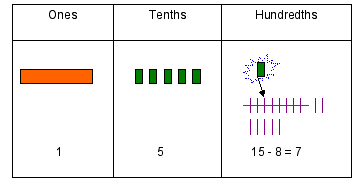I can rename 6 tenths as 5 tenths and ten hundredths. If I trade 1 tenth for 10 hundredths, I have 15 hundredths. 15 hundredths minus 8 hundredths is 7 hundredths.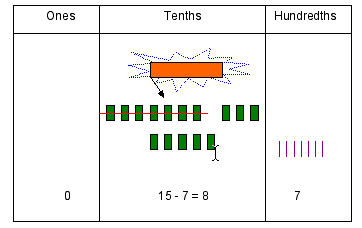Next I move to the tenths. 5 tenths minus 7 tenths I cannot do. I will need to exchange 1 one for 10 tenths, giving me 15 tenths. 15 tenths minus 7 tenths is 8 tenths.I now have 0 ones, 8 tenths and 7 hundredths. 1.65 - 0.78 = 0.87

Example 2: Using place value columns, 2.006 - 0.1 = 1.906

Example 3: Placing extra zeros, 2.7 - 1.342 = 1.358

Example 4: Expert performance of 3.45 - 0.782 = 2.668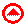Subtraction Quiz

 1 7.8 - 5.9 2 7.004 - 3.8 3 2.1 - 0.954 4 0.813 - 0.3762

Extra questions:

1. Subtract 137.0324 from 138.921

2. What is the difference between 99.99 and 9.999?

3. 271.65 is 19.723 more than a certain number. What is the number?

4. What number is 6.275 less than 10.137?

5. If Meg is 158.3 cm tall and Abdul is 147.9 cm tall, how much taller is Meg than Abdul?

6. How much change will I get if I purchase some bananas for \$4.65 and give the shopkeeper \$20?

If you would like to do some more questions, click here to go to the mixed operations quiz at the end of the division section.